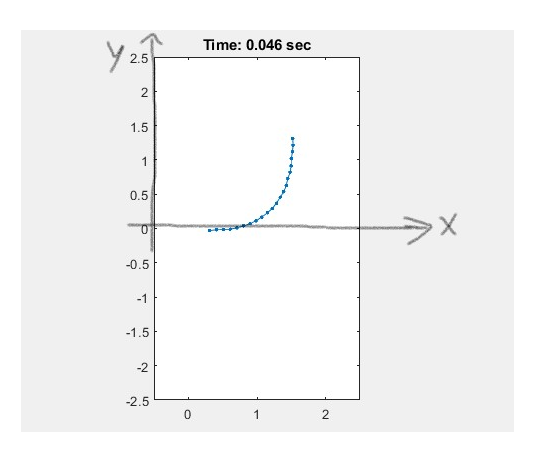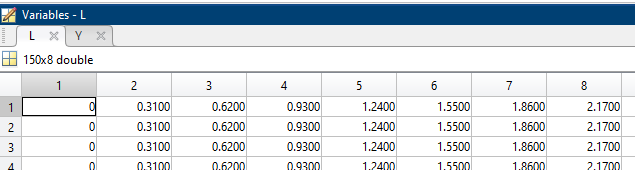## Fluids

Tagged:

•grahamq22
Subscriber
I have data describing motion that I would like to apply to a wall boundary using DEFINE GRID MOTION. This data is x,y,t(time) data. (1*) I can fit a surface to this data outside of ansys and possibly generate some equation of the surface to put into ansys. (2*) However, would I be able to interpolate the data natively in the udf using c to prescribe this motion? (3*) Or lastly, and maybe most likely, is there a way to use a journal file to iteratively update the position of this wall. If not directly, maybe I could get the coordinates of the mesh at each timestep of the motion? then use a journal file to update the mesh like this person has (https://forum.ansys.com/discussion/2878/journal-file-for-dynamic-mesh-update). n
•YasserSelima
Subscriber
Check this post .. it does read a file .. you need to add to this an interpolation functionnhttps://forum.ansys.com/discussion/26049/udf-problem-the-expected-wall-controlled-by-an-external-force-do-not-move n
•grahamq22
Subscriber

Check this post .. it does read a file .. you need to add to this an interpolation functionhttps://forum.ansys.com/discussion/26049/udf-problem-the-expected-wall-controlled-by-an-external-force-do-not-movehttps://forum.ansys.com/discussion/comment/112975#Comment_112975

So I can interpolate it natively? I've only seen examples of people interpolating things like temperature data within UDFs so far. For context, the data is 8 (x,y) ordered pairs varying each time step t, where the x data is equidistant and does not change (only height y changes). The line/wall boundary I want to apply this motion to would have the same length as the range of x, and would lie across the x axis. [frame from animation of line body @ t=0.046]nnSo I would be able to follow steps like in this post (https://www.cfd-online.com/Forums/fluent-udf/176079-fluent-udf-interpolate-material-properties-temp-pressure-dependent-table.html), whereby I read my data with DEFINE_EXECUTE_ON_DEMAND...n... I then write a function to preform a bicubic interpolation, then use DEFINE_PROPERTY to return y (height) continously across the wall's bondary Length/x domain? I assume I would implement DEFINE_GRID_MOTION somewhere here as well.n
•YasserSelima
Subscriber
I did not check the link ... but Don't use define property to return the height. Just define it as static double ...#include udf.hn﻿nstatic double the_height(double t, double x){nn CALCULATE y HERE ..... nnreturn y;nnnDEFINE_GRID_MOTION(.....)n{nn When you need y ...nny = the_height( t, x);nn USE ynnn
•grahamq22
Subscriber

I did not check the link ... but Don't use define property to return the height. Just define it as static double ... #include "udf.h"n﻿nstatic double the_height(double t, double x){nn CALCULATE y HERE .....return y;n}nnDEFINE_GRID_MOTION(.....)n{nn When you need y ...nny = the_height( t, x);nn USE ynn}nhttps://forum.ansys.com/discussion/comment/113164#Comment_113164

Okay, so then CALCULATE y HERE is just the function for interpolating the data.n
•YasserSelima
Subscriber
yes ... read the data by on_demand function and save them in a global variable. And do the interpolation in CALCULATE y HEREnn